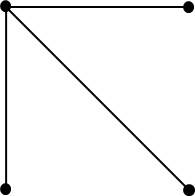## Posts Tagged ‘recursion’

### Computer Science Algorithms are way better than Mathematical Theorems

January 24, 2015

I see a great deal of beauty in mathematics. There is much beauty in a well-described, step-by-step proof of a theorem.

Lately I have been learning algorithms for parsing and compilers from a pair of marvelous books.  I think the author is one of the best technical writers in the world, which makes learning very enjoyable. As I learn the parsing and compiler algorithms described in the books, the same sense of beauty rises up inside me as with a good math theorem proof.

So both Mathematical theorems and Computer Science algorithms have tremendous beauty. Nonetheless, I think that Computer Science algorithms are way better than Mathematical theorems. Here’s why:

In Mathematics there is a tightly constrained framework in which one operates. There is a limited set of building blocks (axioms) and rules that one uses to perform work. Conversely, in Computer Science algorithms there is no such finite, well-defined set of building blocks and rules. Although there are commonly used techniques – recursion, closure, induction, etc. – there are an infinite number of concepts that may be used to create an algorithm. That infinite variety provides enormous power and complexity, and requires great creativity and ingenuity, and is why I think Computer Science algorithms are way better than Mathematical theorems.

 “Parsing Techniques” by Dick Grune et al, “Modern Compiler Design” by Dick Grune et al.

### Learning for the sheer love of it … determining the number of edges in a complete graph

September 7, 2013

As I noted in an earlier blog, even little discoveries makes one feel wonderful. Today I solved a small problem (i.e., made a little discovery) and I feel wonderful.

I am reading a wonderful book, Introduction to Graph Theory. I am at the part where it describes complete graphs. A complete graph is one where each vertex is connected to every other vertex. The author asks, How many edges are in a complete graph with N vertices? I took this as a challenge to use my reasoning skills to figure it out.

Here are three examples of complete graphs and the number of edges in each graph:Let’s arbitrarily choose one vertex. It must connect to the other (n-1) vertices. For example, in the complete graph with 4 vertices, the vertex will connect to the other 3 vertices:The remaining vertices must form a complete graph amongst themselves. Ah Ha! Now we have a simpler problem: find the number of edges in a complete graph of n-1 vertices.

I now switch on my programmer hat. I smell recursion (which I love):

```numEdges (N) {
if (N == 2) then return 1
else return (N-1) + numEdges (N-1)
```

Let’s check that program:

if N = 2, then num edges = 2
if N = 3, then num edges = 2 + 1 = 3
if N = 4, then num edges = 3 + 3 = 6
if N = 5, then num edges = 4 + 6 = 10
if N = 6, then num edges = 5 + 10 = 15
if N = 7, then num edges = 6 + 15 = 21
if N = 8, then num edges = 7 + 21 = 28

Wow! That is cool.

One problem, however. What are the number of edges when, say, N = 1000?

I could implement the above program and set it to solve N=1000. But I know the mathematicians would tell me: Find a formula.

Okay, let’s see … to find the number of edges in a complete graph with N vertices, I must add these numbers:

(N-1) + (N-2) + (N-3) + … + 1

or equivalently:

1 + 2 + … + (N-1)

I remember from school days a formula for the sum of the first N integers:

n(n+1)/2

Of course, I want the sum of the first N-1 integers, so I substitute “n” with N-1:

(N-1)((N-1)+1)/2
= (N-1)(N-1+1)/2
= (N-1)(N)/2
= N(N-1)/2

Let’s check:

if N = 2, then 2(1)/2 = 1
if N = 3, then num edges = 3(2)/2 = 6
if N = 4, then num edges = 4(3)/2 = 6
if N = 5, then num edges = 5(4)/2 = 10
if N = 6, then num edges = 6(5)/2 = 15
if N = 7, then num edges = 7(6)/2 = 21
if N = 8, then num edges = 8(7)/2 = 28

Yea!

I am pretty proud of myself. I solved it, not because it was a homework problem or a work problem. I solved it purely for the love of learning. I figured it out by simple reasoning.

I feel wonderful.